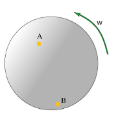# Problem: A merry-go-round is rotating at constant angular speed. Two children are riding the merry-go-round: Ana is riding at point A and Bobby is riding at point B. Part A Which child moves with greater magnitude of velocity? Ana has the greater magnitude of velocity. Bobby has the greater magnitude of velocity. Both Ana and Bobby have the same magnitude of velocity. Part B Who moves with greater magnitude of angular velocity? Ana has the greater magnitude of angular velocity. Bobby has the greater magnitude of angular velocity. Both Ana and Bobby have the same magnitude of angular velocity.

###### FREE Expert Solution

Part A

Velocity:

$\overline{){\mathbf{v}}{\mathbf{=}}{\mathbf{r}}{\mathbf{\omega }}}$

rB is greater than rA. Thus, B has greater magnitude of velocity.

91% (398 ratings)###### Problem Details

A merry-go-round is rotating at constant angular speed. Two children are riding the merry-go-round: Ana is riding at point A and Bobby is riding at point B.Part A Which child moves with greater magnitude of velocity?

1. Ana has the greater magnitude of velocity.
2. Bobby has the greater magnitude of velocity.
3. Both Ana and Bobby have the same magnitude of velocity.

Part B Who moves with greater magnitude of angular velocity?

1. Ana has the greater magnitude of angular velocity.
2. Bobby has the greater magnitude of angular velocity.
3. Both Ana and Bobby have the same magnitude of angular velocity.

Frequently Asked Questions

What scientific concept do you need to know in order to solve this problem?

Our tutors have indicated that to solve this problem you will need to apply the Converting Between Linear & Rotational concept. You can view video lessons to learn Converting Between Linear & Rotational. Or if you need more Converting Between Linear & Rotational practice, you can also practice Converting Between Linear & Rotational practice problems.

What professor is this problem relevant for?

Based on our data, we think this problem is relevant for Professor Kawall's class at UMASS.